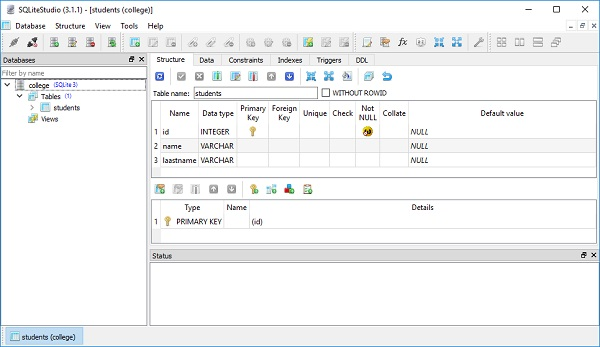# SQLAlchemy Core - Creating Table

Let us now discuss how to use the create table function.

The SQL Expression Language constructs its expressions against table columns. SQLAlchemy Column object represents a column in a database table which is in turn represented by a Tableobject. Metadata contains definitions of tables and associated objects such as index, view, triggers, etc.

Hence an object of MetaData class from SQLAlchemy Metadata is a collection of Table objects and their associated schema constructs. It holds a collection of Table objects as well as an optional binding to an Engine or Connection.

```from sqlalchemy import MetaData
```

Constructor of MetaData class can have bind and schema parameters which are by default None.

Next, we define our tables all within above metadata catalog, using the Table construct, which resembles regular SQL CREATE TABLE statement.

An object of Table class represents corresponding table in a database. The constructor takes the following parameters −

Name Name of the table
Column(s) One or more objects of column class

Column object represents a column in a database table. Constructor takes name, type and other parameters such as primary_key, autoincrement and other constraints.

SQLAlchemy matches Python data to the best possible generic column data types defined in it. Some of the generic data types are −

• BigInteger
• Boolean
• Date
• DateTime
• Float
• Integer
• Numeric
• SmallInteger
• String
• Text
• Time

To create a students table in college database, use the following snippet −

```from sqlalchemy import Table, Column, Integer, String, MetaData

students = Table(
'students', meta,
Column('id', Integer, primary_key = True),
Column('name', String),
Column('lastname', String),
)
```

The create_all() function uses the engine object to create all the defined table objects and stores the information in metadata.

```meta.create_all(engine)
```

Complete code is given below which will create a SQLite database college.db with a students table in it.

```from sqlalchemy import create_engine, MetaData, Table, Column, Integer, String
engine = create_engine('sqlite:///college.db', echo = True)

students = Table(
'students', meta,
Column('id', Integer, primary_key = True),
Column('name', String),
Column('lastname', String),
)
meta.create_all(engine)
```

Because echo attribute of create_engine() function is set to True, the console will display the actual SQL query for table creation as follows −

```CREATE TABLE students (
id INTEGER NOT NULL,
name VARCHAR,
lastname VARCHAR,
PRIMARY KEY (id)
)
```

The college.db will be created in current working directory. To check if the students table is created, you can open the database using any SQLite GUI tool such as SQLiteStudio.

The below image shows the students table that is created in the database −